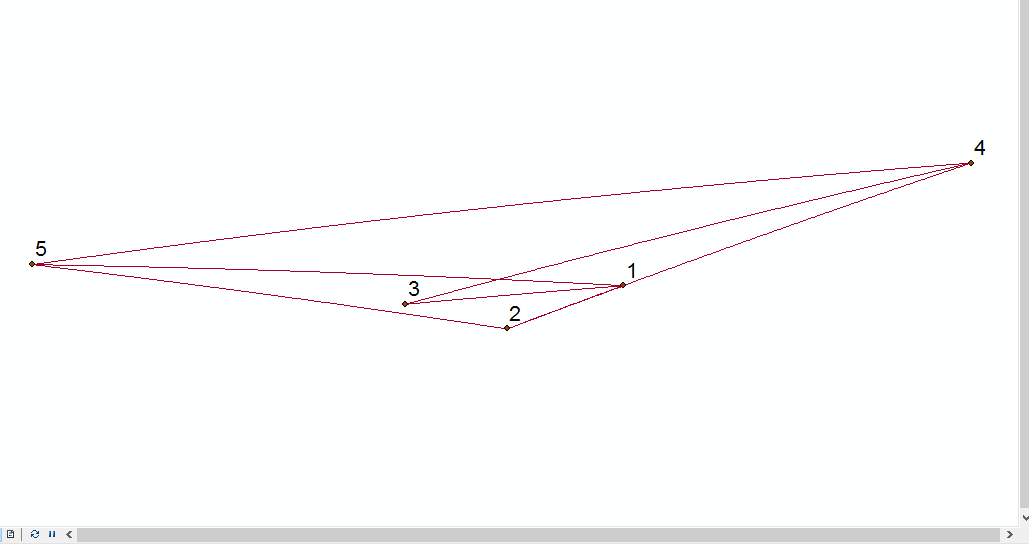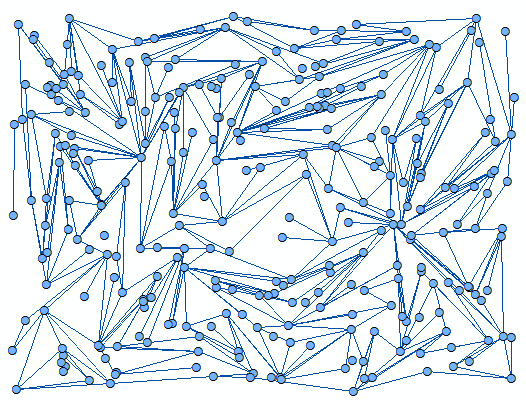# create points and lines both from excel

1184
11
09-27-2016 05:53 PMby
New Contributor III

I have 300 points data with 409 lines connect them all are stored in excel as data. The points are not spatially located, in fact, I can created them using 'create random tool' tool. But when I come to create the lines from the data in excel file, and because the points were created randomly, most of lines cross each other which is not useful to me and needs a lot of work to rearrange the points so that eliminate these crossings when come to create the lines.

I was wondering if there is any way to create these points and lines from excel data avoiding crossing lines as far as can be.

Thanks

11 RepliesbyEsri Esteemed Contributor

Could you elaborate a little more what exactly are you trying to obtain? What is the purpose of this process?

You are using Excel to generate random coordinates. Any other reason for using Excel in this process? Do you exactly need to create 409 lines from 300 points (which do not cross)? If you have image to explain what you need, that would help a lot to understand what you want.by
New Contributor III

Thanks Xander,

Let's say we have oly 5 points created by the tool "create random points". Also, assume we have the lines:

 1 2 1 5 2 5 3 4 3 1 4 5 4 2 4 3

To create the lines, use the tool "xy to lines" and this is what we get:by
New Contributor III

This is only 5 points and 8 lines, and you got a cross between the lines: 1-5 and 3-4 while it is possible to avoid this cross by exchanging the locations of nodes 1 and 3. If there is a way to create these points in a way that if we link the lines we get no cross except the crosses that can not be avoided (in some cases with large system).by
New Contributor III

In summary, you know only the total number of the points and the from/to information about the lines. Nothing else and that is in excel file. Locations of points do not matter.byEsri Esteemed Contributor

So, the objective is to move points and not to create different lines? Because that would be mi idea. Evaluate valid lines between points (using Near) and only add those lines that do not cross other existing lines until the desired number of lines has been achieved. This would require some (python) coding.by
New Contributor III

Exactly, that's what I want to do. If you have any idea with an example would be very helpful. ThanksbyEsri Esteemed Contributor

I don't have an example, but I suppose I can create one. I will see if tonight I can start with it and post back the result here.byEsri Esteemed Contributor

OK, so I created a script that is capable of creating the 300 random points and connect them to 409 lines without intersections of lines. See below the result of two separate runs:andNote that there can be points that do not participate in any line.

The script that created these results is listed below:

``````import arcpy

def main():
import random
import math

arcpy.env.overwriteOutput = True

# featureclasses and sr
fc_pnt = r'C:\GeoNet\RandomPoints2Lines\data.gdb\points'
fc_line = r'C:\GeoNet\RandomPoints2Lines\data.gdb\lines'
sr = arcpy.SpatialReference(3116)

# 409 lines from 300 points
number_lines = 409
number_points = 300
max_diag_cutoff = 0.2 # use 20% of diagonal of extent for max distance between points
min_diag_cutoff = 0.05 # use a minimum dist too

# extent for generating the random points
xmin = 830000
xmax = 838000
ymin = 1180000
ymax = 1186000

# create the random points
print "create the random points"
points = []
dct_pntg = {}
for oid in range(number_points):
x = random.randrange(xmin, xmax)
y = random.randrange(ymin, ymax)
pnt = arcpy.Point(x, y)
pntg = arcpy.PointGeometry(pnt, sr)
points.append(pntg)
dct_pntg[oid] = pntg

# store the random points
arcpy.CopyFeatures_management(points, fc_pnt)

# get diagonal length of extent
diag_length = math.hypot(xmax-xmin, ymax-ymin)
max_dist_allowed = diag_length * max_diag_cutoff
min_dist_allowed = diag_length * min_diag_cutoff # can be set to 0

# create a dct of distances
print "create a dct of distances"
dct_dist = {}
cnt = 0
for oid1, pntg1 in sorted(dct_pntg.items()):
if oid1 % 25 == 0:
print "oid1", oid1
for oid2 in range(oid1, number_points):
cnt += 1
pntg2 = dct_pntg[oid2]
dist = getDistance(pntg1, pntg2)
if all([dist <= max_dist_allowed, dist >= min_dist_allowed]):
oid_key = "{0}#{1}".format(oid1, oid2)
dct_dist[oid_key] = dist

print "number of possible lines:", cnt
print "lines to be considered:", len(dct_dist.keys())

# let's create some lines
print "let's create some lines"
dct_lines = {}
for oid_key, dist in dct_dist.items():
lst_oid = oid_key.split('#')
oid1 = int(lst_oid)
oid2 = int(lst_oid)
polyline = createLine(dct_pntg[oid1], dct_pntg[oid2])
if linesIntersect(dct_lines, polyline, oid_key) == False:
dct_lines[oid_key] = polyline
if len(dct_lines.keys()) >= number_lines:
break

# store the result:
print "store the result"
lines_found = dct_lines.values()
arcpy.CopyFeatures_management(lines_found, fc_line)

def getDistance(pntg1, pntg2):
'''Calculate the distance between two point geometries'''
return pntg1.distanceTo(pntg2)

def createLine(pntg1, pntg2):
'''Create a line from two point geometries'''
sr = pntg1.spatialReference
return arcpy.Polyline(arcpy.Array([pntg1.firstPoint, pntg2.firstPoint]), sr)

def linesIntersect(dct_lines, polyline, oid_key):
'''Verify if lines cross (lines may share start and end points)'''
intersect = False
lst_oid = oid_key.split('#')
oid1 = int(lst_oid)
oid2 = int(lst_oid)
for oid_key2, line in dct_lines.items():
lst_oid2 = oid_key2.split('#')
oid3 = int(lst_oid2)
oid4 = int(lst_oid2)
if all([oid3 != oid1, oid3 != oid2, oid4 != oid1, oid4 != oid2]):
if line.crosses(polyline):
intersect = True
break
return intersect

if __name__ == '__main__':
main()‍‍‍‍‍‍‍‍‍‍‍‍‍‍‍‍‍‍‍‍‍‍‍‍‍‍‍‍‍‍‍‍‍‍‍‍‍‍‍‍‍‍‍‍‍‍‍‍‍‍‍‍‍‍‍‍‍‍‍‍‍‍‍‍‍‍‍‍‍‍‍‍‍‍‍‍‍‍‍‍‍‍‍‍‍‍‍‍‍‍‍‍‍‍‍‍‍‍‍‍‍‍‍‍‍‍‍‍‍‍``````

To run this script you will have to change a number of settings according to your specific needs:

• lines 10 and 11 the output featureclass that will be created for the random points and lines
• line 12 the spatial reference to be used for the output featureclasses
• lines 15 and 16 set the number of points and lines to be created
• lines 17 and 18 set the minimum and maximum lengths of the lines to be created (possibilities to be considered).  Long lines are more probable to have intersections so don't make them too long and perhaps it is not desired that points located directly next to each other are considered in the process
• lines 21 to 24 indicate the extent in which the random points will be created

Try it for yourself and see what the result is. If you have any problems please let me know and we'll see how we can fix it.

Kind regards, Xanderby
New Contributor III

Thank you Xander, appreciate it. just question until I get access to arcgis, the lines you created were based on pre-stored from/to data or arbitrarily? This is important to my work since the lines are pre defined as from-to data and trying avoid overlapping each other as much as possible and of course short line is better desired. Thank you and I will get you back when run it on my data.

Sincerely